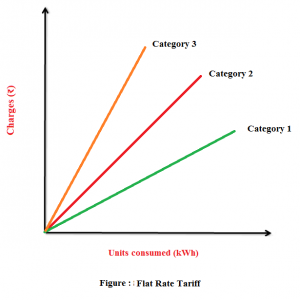# What is the flat rate tariff?

When different types of consumers are charged at different uniform per unit rates, it is said to be flat rate tariff.• In this type, the consumers are grouped into different classes.
• Each class is charged at the different uniform rate.
• The different classes of consumers may be taken into account of their diversity and load factors.
• Since this type of tariff varies according to the way of supply used, separate meters are required for lighting load, power load etc.
• This is probably the most popular tariff structure.
• In flat rate tariff structure, various types of consumers are charged at different according to rate.
• Flat rate for light loads like light, fan are charged a bit higher than loads with heavy consumption.
• The rate at which each category of consumer is to be charged is decided by considering its load factor and power factors into account.

Total Energy Charged = Rs. NU*R

Where,
NU – Number of units (energy) consumed over a billing period.

RFlat rate in rupees per Unit.

Tags: